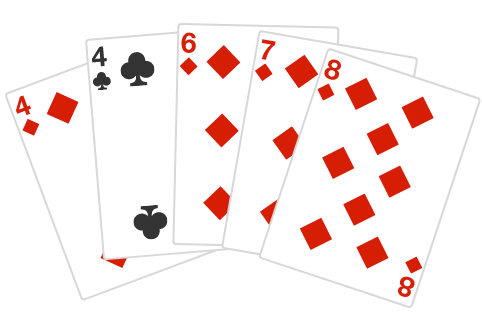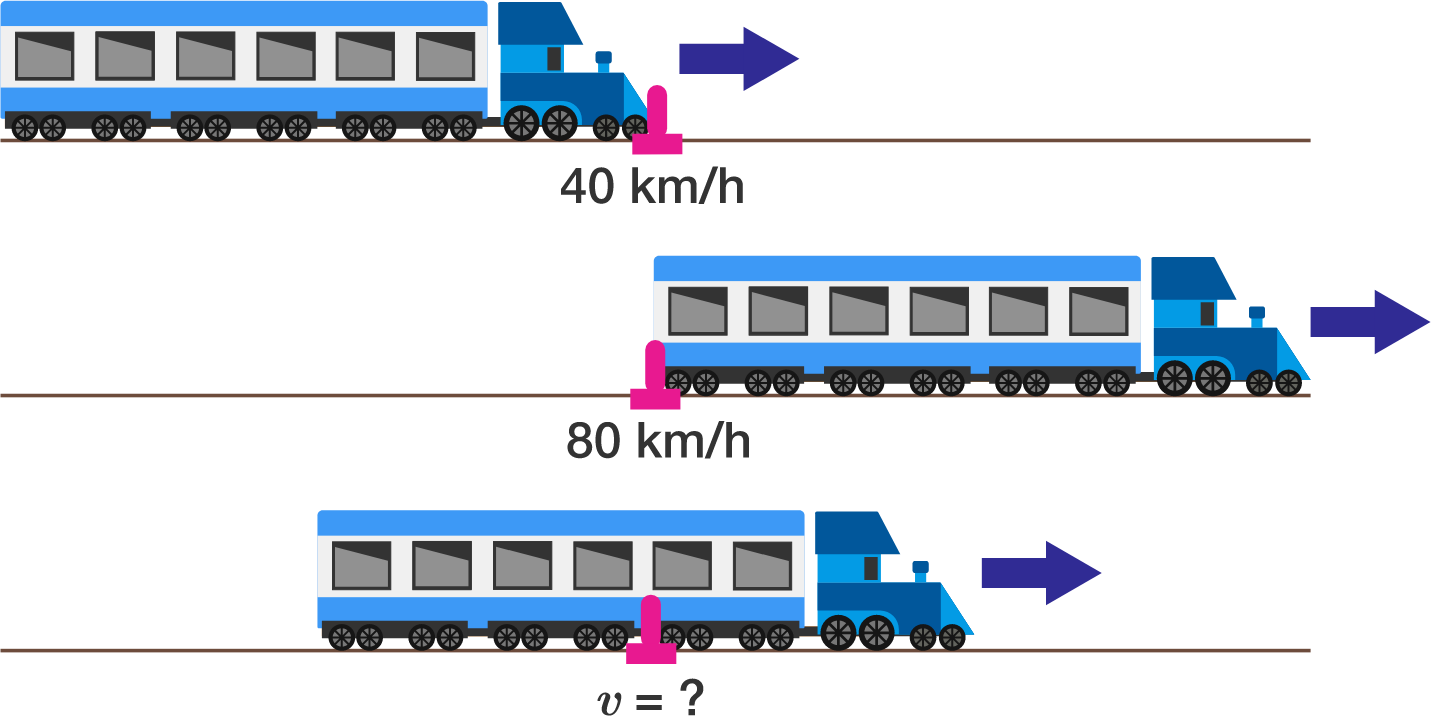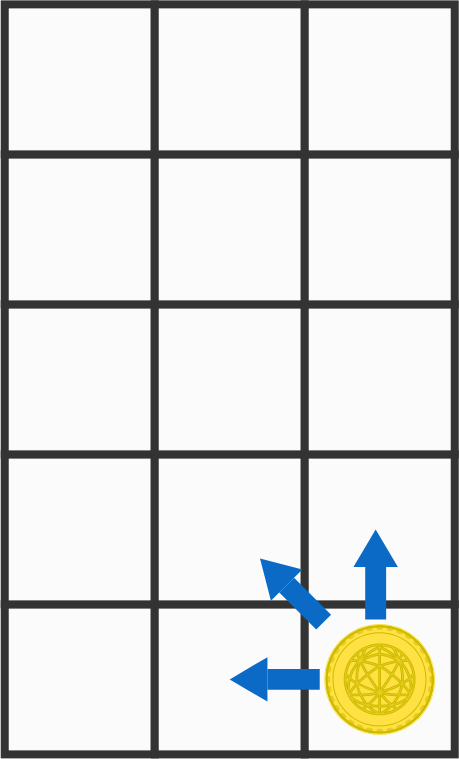# Problems of the Week

Contribute a problem

# 2018-07-30 Basic

How many isosceles triangles are drawn in this regular pentagon?This set of five cards is shuffled together, and then two are randomly drawn, in order.

What is the probability that the second card has a greater number on it than the first?

How many of the first 1000 positive integers contain the digit 0?

A train moving at constant acceleration passes by a pink pole near the track. The front end of the train passes the pole going $40 \text{ km/hr},$ and then the back end of the train passes it going $80 \text{ km/hr}.$

What was the speed at which the middle of the train passed the pole?The coin can only be moved up, left, or diagonally up-and-left.

Charlie and Tracy are playing a game on a $5 \times 3$ grid with a coin in its lower right corner. The rules are as follows:

• Charlie goes first, then they take turns moving the coin.
• The coin can only be moved one space up, left, or diagonally up-and-left.
• The first one to move the coin to the upper left corner wins.

If both play optimally, who will always win?

×